Session 7

Chairman: W. Minkowycz

COMPUTATIONAL RADIATION USING THE FINITE-VOLUME METHOD

G.D. Raithby

Department of Mechanical Engineering

University of Waterloo, Waterloo, Ontario, Canada N2L 3G1

ABSTRACT

The methods described in this paper will be of interest for problems where there is a flowing medium, convective heat transfer, participating-medium radiative heat transfer, and perhaps mass transfer, chemical reaction, and other processes. For such problems, the equations of fluid motion and convective heat transfer, as well as other scalar equations, are often solved using Finite Volume (FV) methods of Computational Fluid Dynamics (CFD). The conversion of the continuous governing equations, for each of these processes, to their respective discrete-equation counterparts is done using a consistent approach. The discrete equations for all variables are solved using the same computational mesh, the same solver , and the same pre- and post-processor tools are used. On the other hand, radiation is often treated as another kind of process, and the distinctly different approaches to solving the equations of radiant heat transfer reflect these differences. The result is a radiation solver that is often not integrated with the solver for the other quantities. The underlying motivation for the FV method 1,2 (presented in this paper) is to treat radiation consistently with other conserved quantities, so that its calculation fits seamlessly with the solution of other variables. That is, the same discretization method is applied, and the equations are solved using the same code (i.e. the same spatial grid, solver, and processor tools are used).

Modern FV methods can be used with an unstructured grid. An important property of any CFD method is that the exact solution of the equations is approached as the computational mesh is refined. The distinctive property that makes the FV method particularly attractive is that exact conservation of each conserved variable is ensured for each discrete volume. Besides the properties already listed, the FV radiation method must also have these properties.

The Radiation Transfer Equation expresses conservation of radiant energy, and the dependent variable in this equation is the intensity. Intensity is a six-dimensional variable, depending on position in space (three dimensions), on direction (two dimensions) and on wavelength. If the radiation is treated as gray, as it is in the paper, then the dimensionality decreases to five. The starting point of any FV implementation is the integral conservation equations, where the integration is over a discrete interval of all dependent variables. For radiation, integration is over the three space dimensions (i.e. over a discrete spatial volume) and over a discrete interval of direction (i.e. over a solid angle). Taken together, the spatial volume and solid angle represent a hypervolume. It is the integration over a discrete solid angle that makes the FV method distinct from the Discrete Ordinates Method 3.

From the integral equation just described, a discrete equation for intensity is derived. There is one such equation for each hypervolume (that is one for each combination of spatial volume and solid angle). The spatial approximations are consistent with those employed for other conserved variables. Over a discrete solid angle, the approximation that intensity is constant is introduced.

The present paper elaborates on the description of the FV method published earlier1,2 1,2. Results are also presented for one-dimensional, two-dimensional, and axisymmetric problems which involve absorption/emission and scattering (both isotropic and anisotropic) within simple enclosures (where other solutions are available for comparison). These examples show that accuracy obtained from the solution of the discrete equations increases quadratically as the solid angle is decreased, and that quite accurate predictions are obtained with a relatively coarse angular grid. It is also demonstrated that the solution for radiant intensity requires an increase in the number of iterations as the strength of the medium participation increases; a correction method, presented earlier4, is described which greatly reduces the number of iterations.

Predictions are also provided for more complex three-dimensional problems. Radiant transport is predicted in a complex enclosure with internal obstacles. The medium is assumed to be non-participating so that results from a surface-to-surface code can be used for comparison. Predictions of collimated radiation in the same geometry are also provided.

Most methods that are applicable to participating-medium radiation are applied to regular geometries, where the surfaces of the enclosure coincide with coordinate surfaces. This paper provides applications of the FV method to enclosures where the surfaces have arbitrary orientation. An analysis is provided of the loss of accuracy that results from arbitrary surface orientation.

REFERENCES

1. Raithby, G.D. and Chui, E.H., A Finite-Volume Method for Predicting Radiant Heat Transfer in Enclosures with Participating Media, J. Heat Transfer, Vol. 112, pp. 415-423, 1990.
2. Chai, J.C., Parthasarathy, G., Lee, H.S., and Patankar, S.V., Finite Volume Radiation Heat
3. Transfer Procedure for Irregular Geometries, J. Thermophysics and Heat Transfer, Vol. 9, No. 3, pp. 410-415, 1995.
4. Fiveland, W.A. and Jessee, J.P., Finite Element Formulation of the Discrete-Ordinates Method for Multidimensional Geometries, J. Thermophisics and Heat Transfer, Vol. 8, No. 3, pp. 426-433, 1994
5. Chui, E.H. and Raithby, G.D., Implicit Solution Scheme to Improve Convergence Rate in Radiative Transfer Problems, Numerical Heat Transfer, Part B, Vol. 22, pp.251-272, 1992.

NUMERICAL ANALYSIS OF RADIATIVE HEAT FLUX ON WALLS OF THE COMBUSTION CHAMBER IN A DIESEL ENGINE

Jerzy Banaszek, Piotr Furmanski, Tomasz S. Wisniewski

Institute of Heat Engineering, Warsaw University of Technology,

ul. Nowowiejska 25, 00-665 Warszawa, Poland

Radiation heat transfer in a diesel engine is believed to have a substantial share in the global heat transfer in the engine. It is specially important during the combustion period of the engine cycle, when depending on the engine type, it is estimated to be responsible for up to 40 percent of the engine heat losses. Despite the well-known estimates of radiation share in the global heat transfer, space distribution of radiative flux on walls of the combustion chamber is not known and can be an important factor of thermal load of some parts of the engine1-2.

A method of determination of distribution of the radiative heat flux, based on two-zone thermodynamic model and integral equation of radiative heat transfer, has been proposed by Furmañski et al.3 In the case of axisymmetric geometry of the combustion chamber they have assumed that the burnt zone has a form of a cylinder described by a radius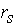and a height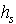(Fig.1) and they have derived the following integral equation for the radiative heat flux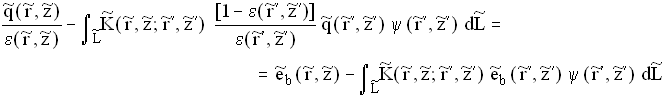(1)

where the Kernel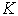is a complicated integral taking into account shading of some surfaces by others.

The main objective of this paper is to develop a numerical scheme for solution of the above integral equation. The integral equation, that can be rewritten in a more compact form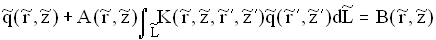, (2)

has been solved numerically by the use of the weighted residual approach, i.e.: the point collocation method, along with the local piece-wise interpolation technique, inherent in the Finite Element Method (FEM) and Boundary Element Method (BEM).

The real boundary surfaces of the combustion chamber (walls A,B,C,D,E and F in Fig.1) as well as the imaginary boundaries of the developing burnt zone (surfaces G,H and I in Fig.1) have been first discretized into irregular one-dimensional linear or parabolic finite elements4. The number of these subdomains has been varied with the temporary volumes of both the chamber and the burn zone, dependent on the actual crank angle, in accordance with the rule that all finite elements are of a comparable length. Within each element the unknown radiative heat flux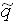has been interpolated in terms of the sum of products of its nodal values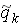and relevant linear or parabolic Lagrange polynomials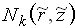4: On putting the element-wise interpolation of the unknown radiative heat flux into the integral equation, one obtains the residuum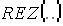- an error of the integral equation caused by the fact that the radiative flux distribution given by the above equation is not exact but rather approximate: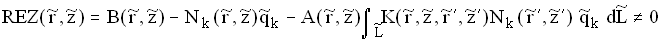(3)

As the residuum does not vanish for any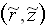, the weighted residual technique (WRM) has to be used, where the error is minimized by weighting the residual value over the whole domain, through the weighting functions Wk defined in the local element basis: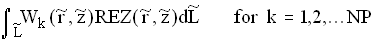.

where NP is the number of interpolation nodes along the whole boundary.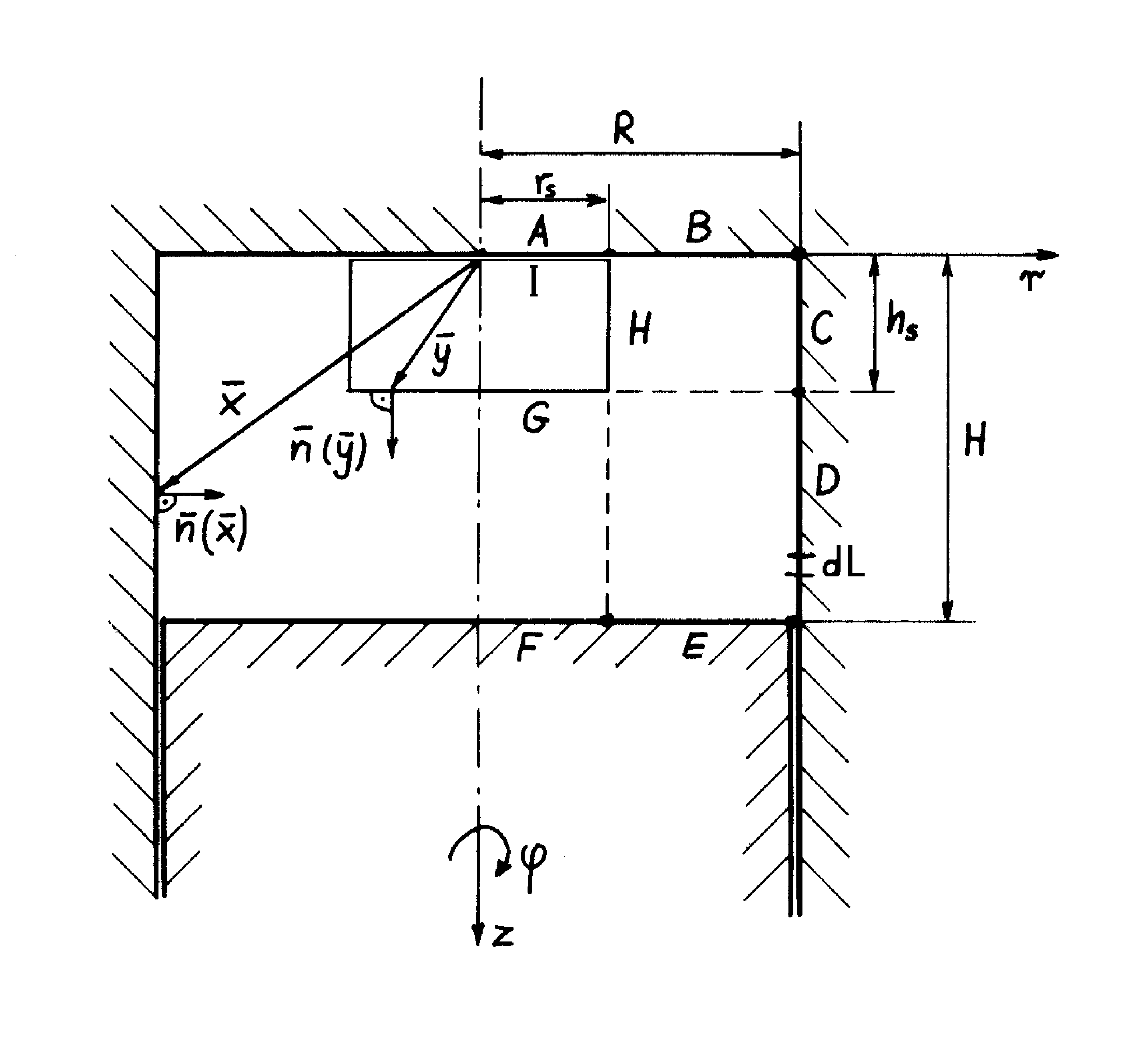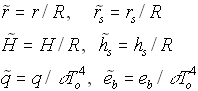where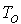is a certain reference temperature.

Fig.1 A scheme of the combustion chamber and the burnt zone.

The point collocation method, where the function Wk is given by the Dirac's delta distribution, has been applied here. Thus, the integral equation is replaced by the set of NP algebraic equations where the individual equation for the collocation point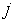, that coincides with the node of the finite element grid, has the form of Eq.(1) with. The piece-wise interpolations enable to determine integrals of these equations by summing shares of element integrals. Each of them has been calculated by the Gaussian quadrature. The kernel functionis obtained by integration over the angle of sight. In this case, the integral has been calculated by the use of the adaptive technique where the accuracy of integration (performed by Simpson's rule and Romberg extrapolation) is adjusted to the behaviour of the integrand4.

Radiative heat flux governed by the integral equation depends on temperatures on all surfaces that are not in a shadow zone. In consequence, a matrix of the resulting set of algebraic equations is fully populated and its pivots are not necessary the biggest entries of the matrix rows. Therefore, the set of the above algebraic equations has been solved by the direct Gauss elimination method with preliminary scaling (the operation of adjusting the coefficients of a set of eqs so that they are all of the same order of magnitude) and partial pivoting4 to avoid zeros in the diagonal position during the elimination process and to diminish the round-off error.

Fig.2 shows the radiative heat flux distribution along the combustion chamber walls for two different crank angles (from the range of -120¸ 680). The temporary dimension-less height of the chamber (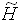), as well as the radius () and height (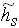) of the cylindrical burnt zone are also given. These preliminary results show that the radiative heat flux distribution on the wall of the chamber can be very uneven with the peak values greatly exceeding the mean values. This fact indicates that different parts of the engine chamber can be differently thermally loaded.

The paper presents only the preliminary analysis of the problem. Next step will be its extension to the case of the partially transparent burnt zone.

ACKNOWLEDGEMENT

This work was supported by the National Committee for Scientific Research (grant No 0442/ S6/ 94/06).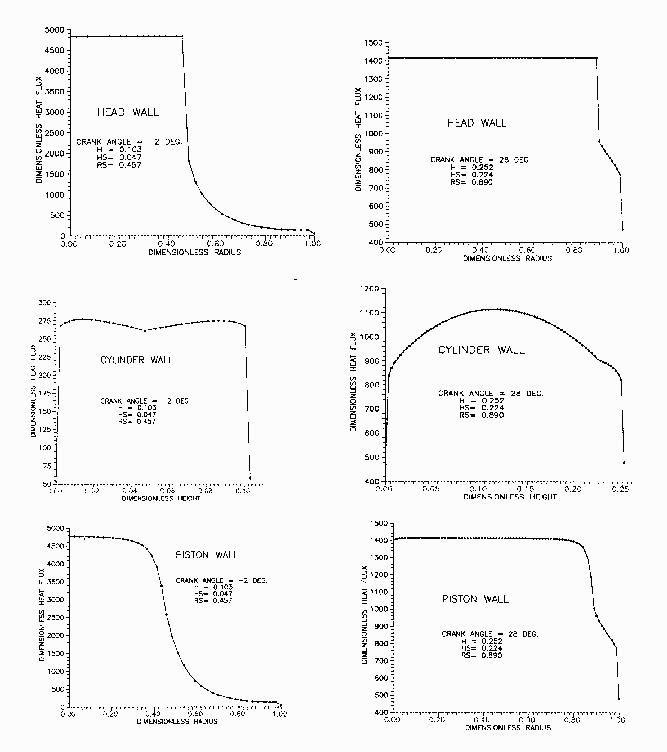Fig.2. Dimensionless heat flux distribution on walls of the combustion chamber for two different values of the crank angle (assumed wall emissivity is).

REFERENCES

1. Menguc, M.P., Viskanta, R. and Ferguson C.R., Multidimensional modeling of radiative heat transfer in diesel engines, SAE Paper No. 850503, pp.728-745, 1985.
2. Morel, T. and Keribar, R., Heat Radiation in D.I. Diesel Engines, SAE Paper No 860445, pp.1141- 1157, 1986.
3. Furmanski, P., Banaszek, J, and Winiewski, T., Radiative Heat Flux Distribution on Walls of the Combustion Chamberin Diesel Engine. Opaque Burnt Gas Zone, Journal of KONES, Vol.2,
pp.69-76, 1996.
4. Gerald, C.F. and Wheatly, P.O., Applied Numerical Analysis, Addison-Wesley Publishing Company, New York 1989.